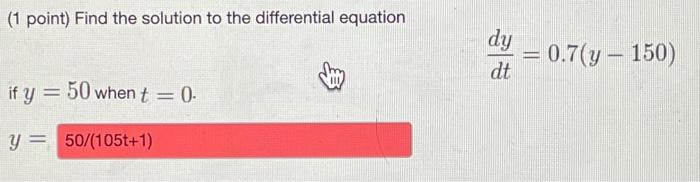# Question (1 point) Find the solution to the differential equation dy = 0.7(y – 150) - dt if y = 50 when t = 0. = y = 50/(105t+1)